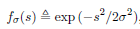# BPDN l0-norm approximation

Hi to everyone!I want to solve the BPDNBut instead of using a sparsity inducing l1-norm …… i want to use this approximation function (please replace the x from above by s, sorry):whereandm denotes the number of elements in s.

I assumed this problem to be convex, and therefore tried to implement it directly using this expression:

minimize(-sum(sigma * inv_pos(pow_abs(s,2) + 2 * power(sigma,2))))

But this does not work (“Disciplined convex programming error”). What needs to be changed in this formulation? I cant see what’s wrong since the problem must be convex?